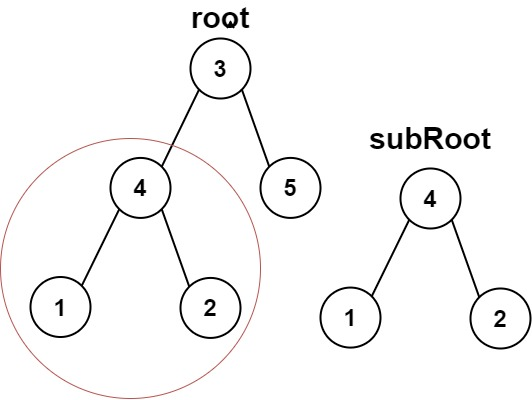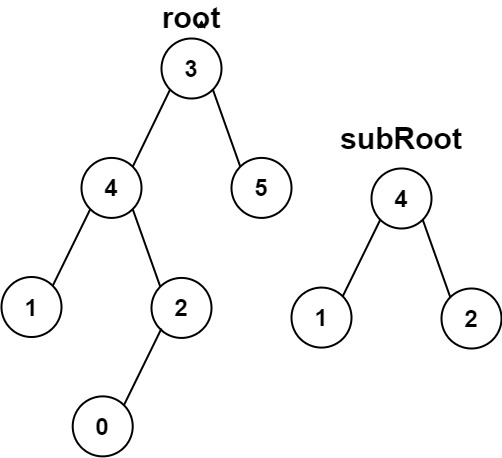# 572. Subtree of Another Tree ⭐

Medium
Given the roots of two binary trees `root` and `subRoot`, return `true` if there is a subtree of `root` with the same structure and node values of` subRoot` and `false` otherwise.
A subtree of a binary tree `tree` is a tree that consists of a node in `tree` and all of this node's descendants. The tree `tree` could also be considered as a subtree of itself.
Example 1:Input: root = [3,4,5,1,2], subRoot = [4,1,2]
Output:
true
Example 2:Input: root = [3,4,5,1,2,null,null,null,null,0], subRoot = [4,1,2]
Output:
false
Constraints:
• The number of nodes in the `root` tree is in the range `[1, 2000]`.
• The number of nodes in the `subRoot` tree is in the range `[1, 1000]`.
• `-104 <= root.val <= 104`
• `-104 <= subRoot.val <= 104`

### 解題

/**
* Definition for a binary tree node.
* type TreeNode struct {
* Val int
* Left *TreeNode
* Right *TreeNode
* }
*/
func isSubtree(root *TreeNode, subRoot *TreeNode) bool {
if root == nil {
return false
}
if isSame(root, subRoot) { return true }
return isSubtree(root.Left, subRoot) || isSubtree(root.Right, subRoot)
}
func isSame(root *TreeNode, subRoot *TreeNode) bool {
if root == nil && subRoot == nil { return true }
if root == nil || subRoot == nil { return false }
if root.Val != subRoot.Val { return false }
return isSame(root.Left, subRoot.Left) && isSame(root.Right, subRoot.Right)
}